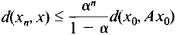# Contraction Mappings, Principle of

The following article is from The Great Soviet Encyclopedia (1979). It might be outdated or ideologically biased.

## Contraction Mappings, Principle of

a fundamental principle of the theory of metric spaces concerning the existence and uniqueness of a fixed point of a set under some special (“contracting”) mapping of the set into itself. The principle of contraction mappings is made use of primarily in the theory of differential and integral equations.

Let us consider an arbitrary mapping A of a metric space M into itself that associates with each point x in M some point y = Ax in M. This mapping gives rise in M to the equation

(*) Ax = x

The effect of the mapping A on x can be interpreted as a displacement of x to the point y = Ax. The point x is called a fixed point of A if it is a solution of equation (*). Thus, the problem of the solvability of equation (*) is equivalent to the problem of finding the fixed points of A.

A mapping of a metric space M into itself is said to be a contraction mapping if there exists a positive number α < 1 such that for any points x and y in M

d(Ax, Ay) ≤ αd(x, y)

where d(u, v) denotes the distance between the points u and v of M.

The principle of contraction mappings asserts that every contraction mapping of a complete metric space into itself has one and only one fixed point. Moreover, for any initial point x0 in M, the sequence {xn} defined by the recurrence relations

xn = Axn–1n = 1,2,…

has as its limit the fixed point x of A. The following estimate for the error can be made:The principle of contraction mappings can be used to prove important existence and uniqueness theorems dealing with solutions of differential, integral and other equations. When the principle of contraction mappings is applicable, a solution can be computed to any desired accuracy by means of the method of successive approximations.

After a definite choice of the complete metric space M and the construction of the mapping A, these problems reduce first to equation (*). Conditions are then found under which A is a contraction mapping.

### REFERENCE

Smirnov, V.I. Kurs vysshei matematiki, vol. 5. Moscow, 1959.

SH. A. ALIMOV

The Great Soviet Encyclopedia, 3rd Edition (1970-1979). © 2010 The Gale Group, Inc. All rights reserved.
Site: Follow: Share:
Open / Close